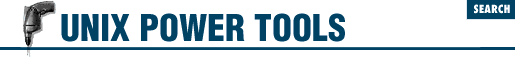home | O'Reilly's CD bookshelfs | FreeBSD | Linux | Cisco | Cisco Exam

#Chapter 49Working with Numbers## 49.4 bc's Sine and Cosine Are in Radians

While using the bc utility the other day, I had the occasion to use the arbitrary precision math library, which is the option -l (lowercase letter "l") of this utility. I wanted to do a simple computation involving the sine of an angle.

I assumed , after reading the manual page, that all I had to do was put in the angle (in degrees) in the sine function. For example, the sine of 30 degrees would be given by:

``````

s(30)
```
```

However, this didn't work. A little investigation revealed that the angle shouldn't be in degrees but in radians. For example, the sine of 30 degrees or 0.5263 radians,

``````

s(.5263)
```
```

gives the correct answer of 0.5.

This is also true of the cosine function.

- MS in comp.unix.questions on Usenet, 19 April 198949.3 Gotchas in Base Conversion49.5 Base Conversion Using cvtbase